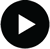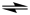## Balancing Chemical Equations### Chemical and Ionic EquationsVideo tutorial
A chemical equation describes what happens when a chemical reaction takes place. It uses chemical formulae to tell us what happens when the reactants (left) react to form the products.

d For example, when methane burns in air, the reactants are CH4(g) (methane) and O2(g) (oxygen in the air). The products are CO2(g) (carbon dioxide) and H2O(g) (water). An arrow meaning react to produce is used to show the process:
CH4(g) + O2(g)CO2(g) + 2H2O(l)
The arrow tells us that methane and oxygen react to form carbon dioxide and water. The reverse reaction, in which carbon dioxide and water react to form methane and oxygen does not occur so the arrow only points from left to right. Some reactions, however, can go either way. For example, N2(g) (nitrogen) and H2(g) (hydrogen) react to form NH3(g) (ammonia). However NH3(g) will also react with itself to form N2(g) and H2(g). Such reactions are said to be reversible. This is represented by either a double headed arrow, ↔, or the equilibrium arrow, ⇌:
 N2(g) + 3H2(g) ↔ 2NH3(g) or N2(g) + 3H2(g)2NH3(g)